# 我们的数据集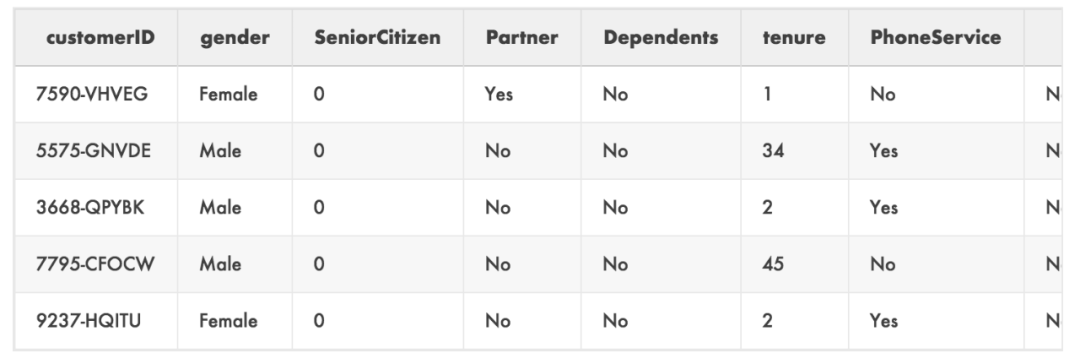dummies = pd.get_dummies(
data[[ 'gender', 'SeniorCitizen', 'Partner', 'Dependents', 'tenure', 'PhoneService', 'MultipleLines', 'InternetService', 'OnlineSecurity', 'OnlineBackup', 'DeviceProtection', 'TechSupport', 'StreamingTV', 'StreamingMovies', 'Contract', 'PaperlessBilling', 'PaymentMethod', 'Churn' ]]
)
data = dummies.join(data[['MonthlyCharges', 'TotalCharges']])


data['TotalCharges'] = data[['TotalCharges']].replace([' '], '0')
data['TotalCharges'] = pd.to_numeric(data['TotalCharges'])


from matplotlib import pyplot as plt
plt.scatter( data['tenure'], data['MonthlyCharges'], c=data['Churn_Yes'])
plt.xlabel('Customer Tenure (Months)')
plt.ylabel('Monthly Charges')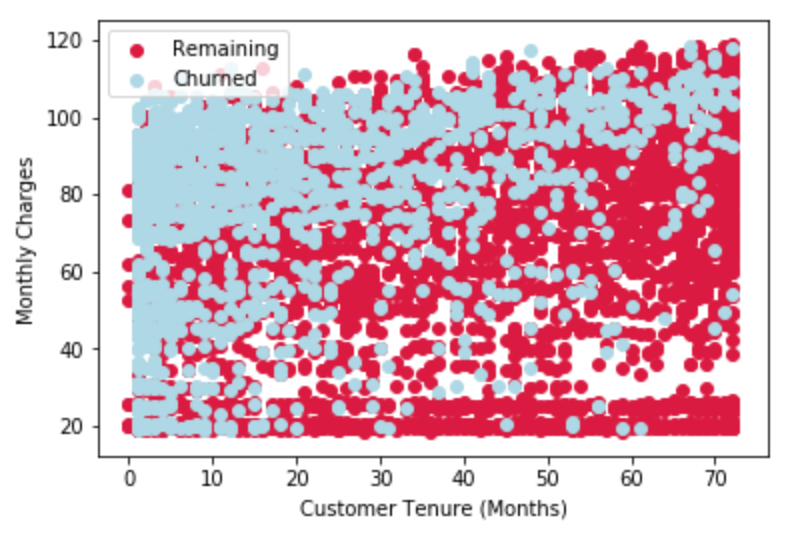# 逻辑回归

from sklearn.linear_model import LogisticRegression
from sklearn.model_selection import train_test_split
from sklearn.metrics import confusion_matrix, accuracy_score
X_train, X_test, y_train, y_test = train_test_split(data[x_select], data['Churn_Yes'])
clf = LogisticRegression(solver='lbfgs', max_iter=1000) clf.fit(X_train, y_train)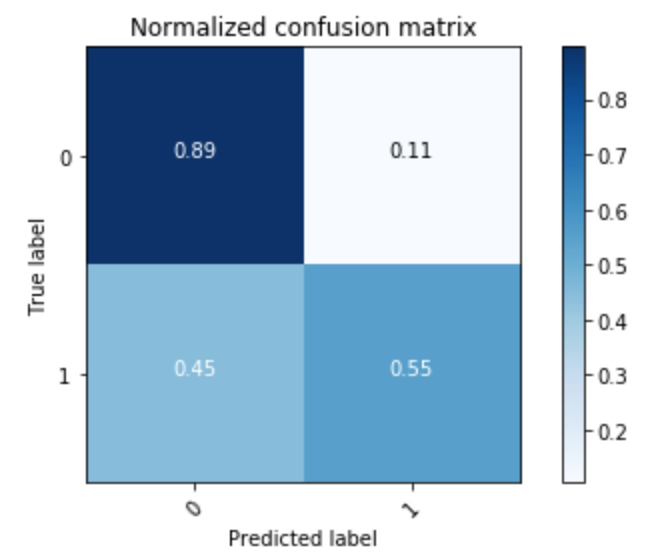# Cox比例风险模型

Cox PH模型是一种基于回归的模型，它分析数据集关于病人(或客户)生存时间的协变量(特征)。它被称为比例风险模型，因为它描述了每个特征如何在基线存活率上按比例增加风险的。

# 使用CoxPH模型

1. “age”(病人开始服药和最近一次观察他们的状态之间的时间差异，在我们的例子中，为顾客加入服务的时间和最近一次观察他们是否流失之间的时间差异)

2. “event”(表示事件是否发生的二进制标志，如死亡或流失)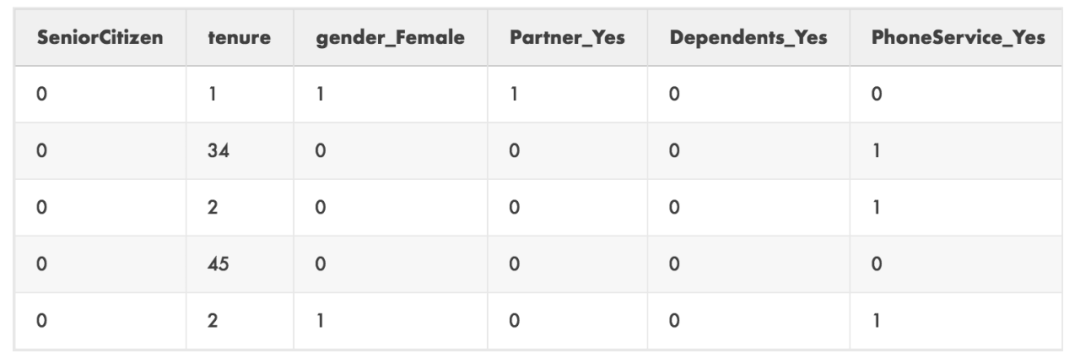from lifelines import CoxPHFitter

cph_train, cph_test = train_test_split(data[x_select], test_size=0.2)
cph.fit(cph_train, 'tenure', 'Churn_Yes')


lifelines包有一些独特之处，可能会给使用惯了Scikit-Learn的用户带来一些麻烦，首先，包含churn的列需要包含在传递给fit调用的数据集中。因此，我们不能像在逻辑回归中那样将数据集分成四份(对X和y进行训练和测试)，我们必须将其分成两份。这与R语法非常相似，你可以在单个数据集中指定相关的列，而算法会负责将它们从训练数据中删除。

cph.fit调用的时候，你需要传入三个不同的参数。第一个是我们使用train_test_split创建的数据集，第二个是‘age’列(在我们的例子中是tenure)，第三个是'event'列(在我们的例子中是Churn_Yes)。

lifelines包的下一个独特之处是可以在模型上使用的.print_summary方法(另一个从R借鉴的方法)。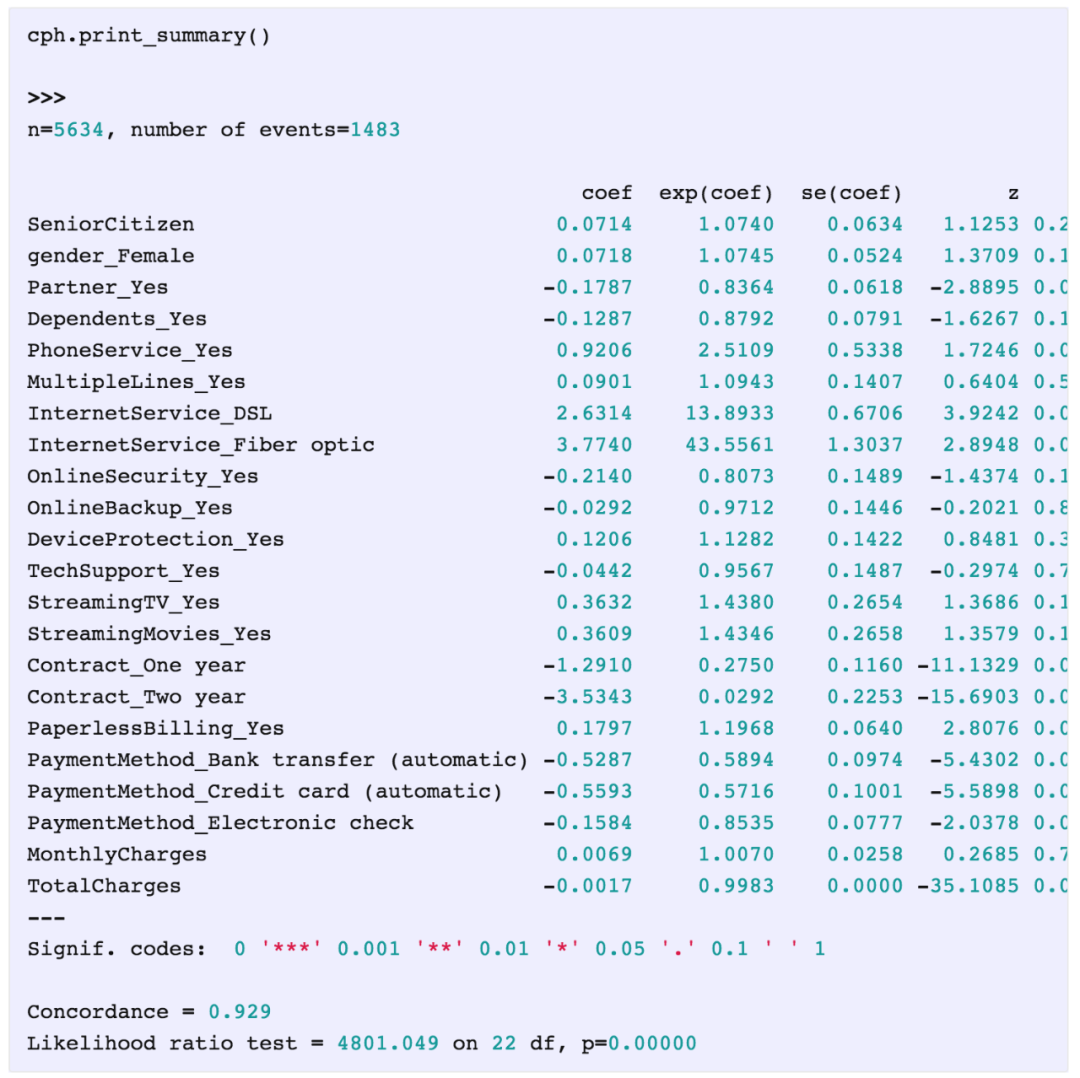1. 我们可以看到在输出的顶部列出了n=5634的样本数量，在其旁边是我们的event数量(流失的客户)。

2. 我们得到了模型的系数。这些是非常重要的，它们告诉我们每个特征是如何“增加”风险的，因此，如果系数是一个正数，那么该特征使客户更有可能流失，如果是负数，那么拥有该特征的客户就不太可能流失。

3. 我们可以得到特征的显著性值，一个非常好的补充！

4. 我们得到了一致性。

# Cox模型绘图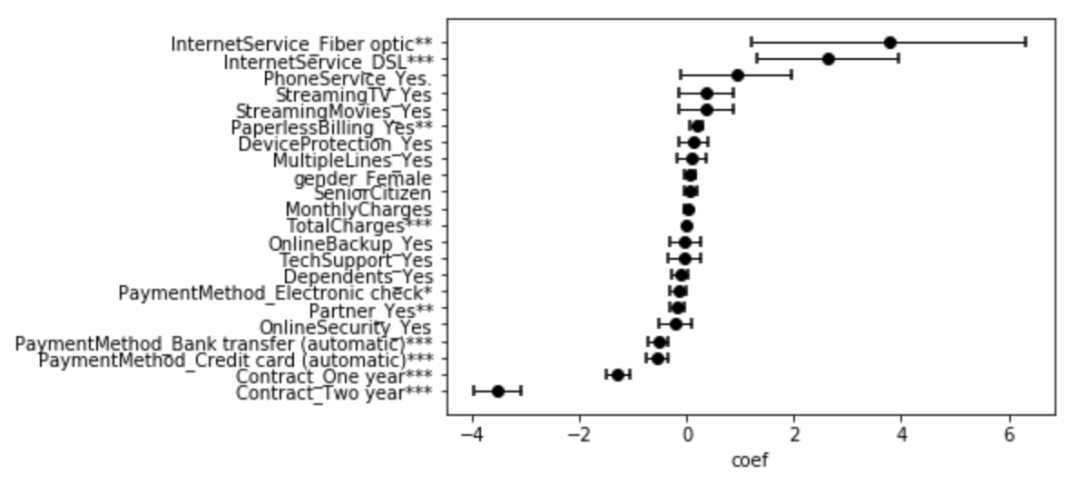cph.plot_covariate_groups('TotalCharges', groups=[0,4000])


.plot_covariate_groupslifelines 中的一个方法，第一个输入是特征的名字，第二个是组的范围。所以，这里我们看的是客户的Total Charges接近0以及Total Charges接近4000的生存曲线的对比，看起来是这样：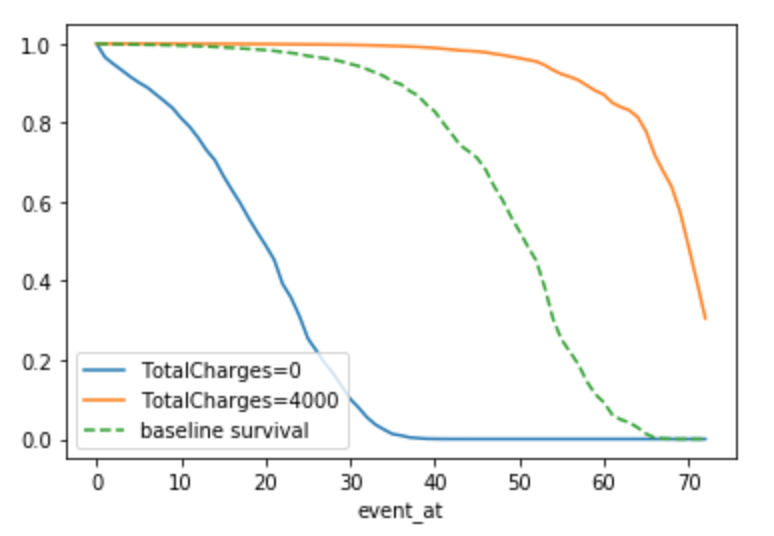# 流失预测

censored_subjects = data.loc[data['Churn_Yes'] == 0]


unconditioned_sf = cph.predict_survival_function(censored_subjects)


conditioned_sf = unconditioned_sf.apply(lambda c: (c / c.loc[data.loc[c.name, 'tenure']]).clip_upper(1))



subject = 12
unconditioned_sf[subject].plot(ls="--", color="#A60628", label="unconditioned")
conditioned_sf[subject].plot(color="#A60628", label="conditioned on $T>58$") plt.legend()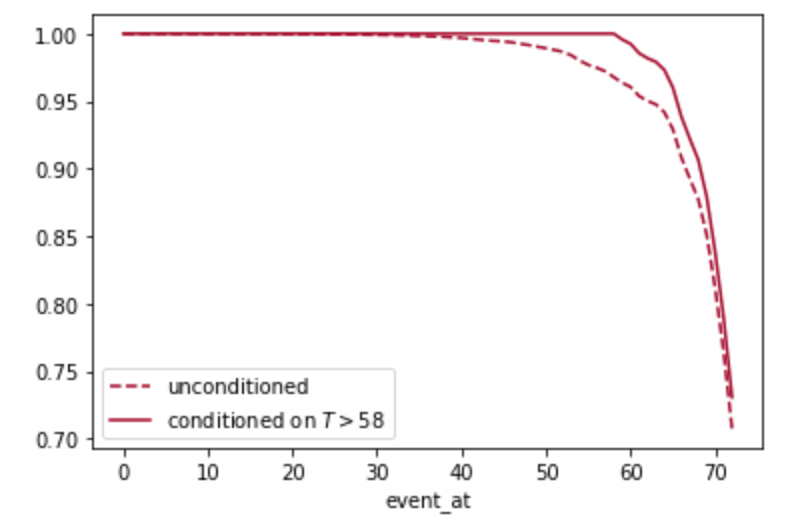from lifelines.utils import median_survival_times, qth_survival_times

predictions_50 = median_survival_times(conditioned_sf)
# This is the same, but you can change the fraction to get other
# %tiles.
# predictions_50 = qth_survival_times(.50, conditioned_sf)



values = predictions_50.T.join(data[['MonthlyCharges','tenure']])
values['RemainingValue'] = values['MonthlyCharges'] * (values[0.5] - values['tenure'])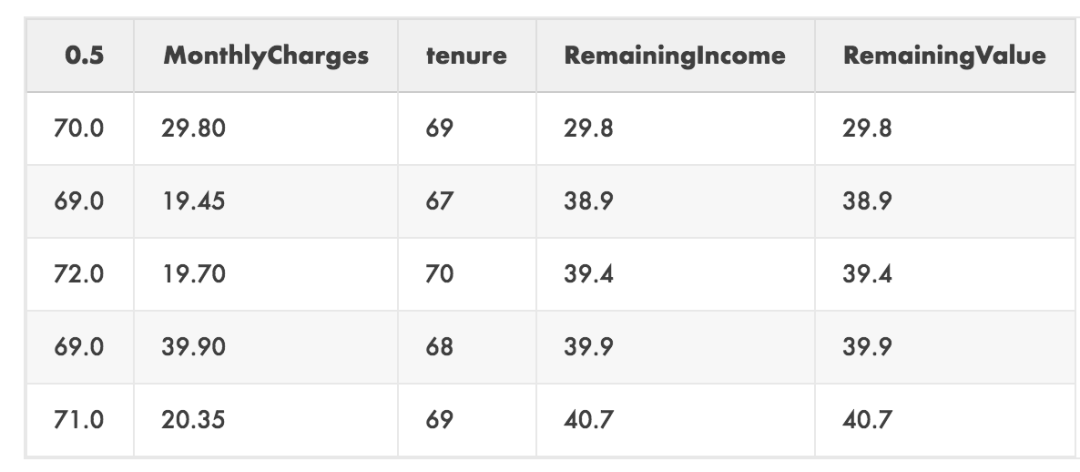# 流失预防

1. 有2年的合同

2. 有一年的合同

3. 用信用卡付款

4. 银行转帐付款

upgrades = ['PaymentMethod_Credit card (automatic)', 'PaymentMethod_Bank transfer (automatic)', 'Contract_One year', 'Contract_Two year']

results_dict = {}

for customer in values.index:
actual = data.loc[[customer]] change = data.loc[[customer]]
results_dict[customer] = [cph.predict_median(actual)]
results_dict[customer].append(cph.predict_median(change))

results_df = pd.DataFrame(results_dict).T
actions = values.join(results_df).drop([0.5], axis=1)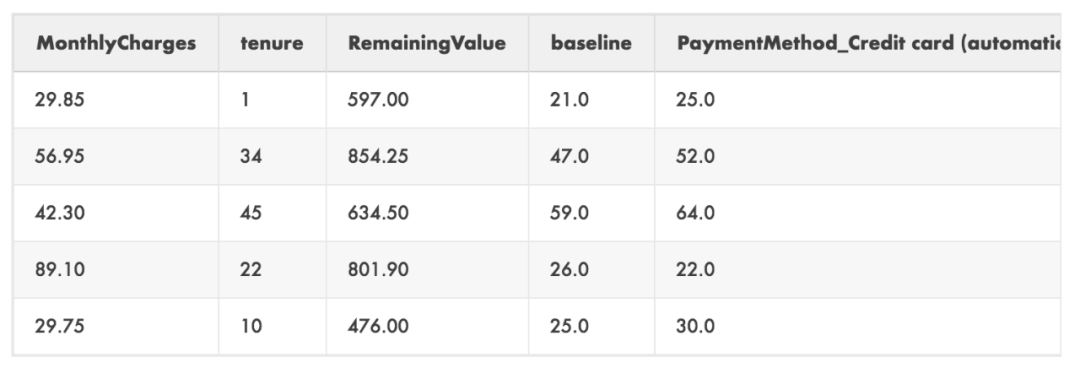actions['CreditCard Diff'] = (
actions['PaymentMethod_Credit card (automatic)'] -
actions['baseline']
) * actions['MonthlyCharges']

actions['BankTransfer Diff'] = (
actions['PaymentMethod_Bank transfer (automatic)'] -
actions['baseline']
) * actions['MonthlyCharges']

actions['1yrContract Diff'] = (
actions['Contract_One year'] - actions['baseline']
) * actions['MonthlyCharges']

actions['2yrContract Diff'] = (
actions['Contract_Two year'] - actions['baseline']
) * actions['MonthlyCharges']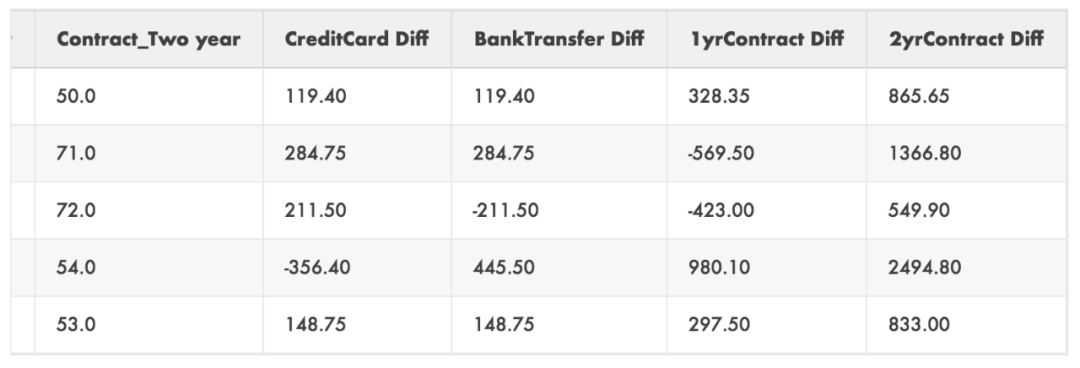# 准确率以及校准

from sklearn.calibration import calibration_curve

plt.figure(figsize=(10, 10))

ax1 = plt.subplot2grid((3, 1), (0, 0), rowspan=2)
ax1.plot([0, 1], [0, 1], "k:", label="Perfectly calibrated")

probs = 1-np.array(cph.predict_survival_function(cph_test).loc)

actual = cph_test['Churn_Yes']

fraction_of_positives, mean_predicted_value = \
calibration_curve(actual, probs, n_bins=10, normalize=False)

ax1.plot(mean_predicted_value, fraction_of_positives, "s-", label="%s" % ("CoxPH",))

ax1.set_ylabel("Fraction of positives")
ax1.set_ylim([-0.05, 1.05]) ax1.legend(loc="lower right")
ax1.set_title('Calibration plots (reliability curve)')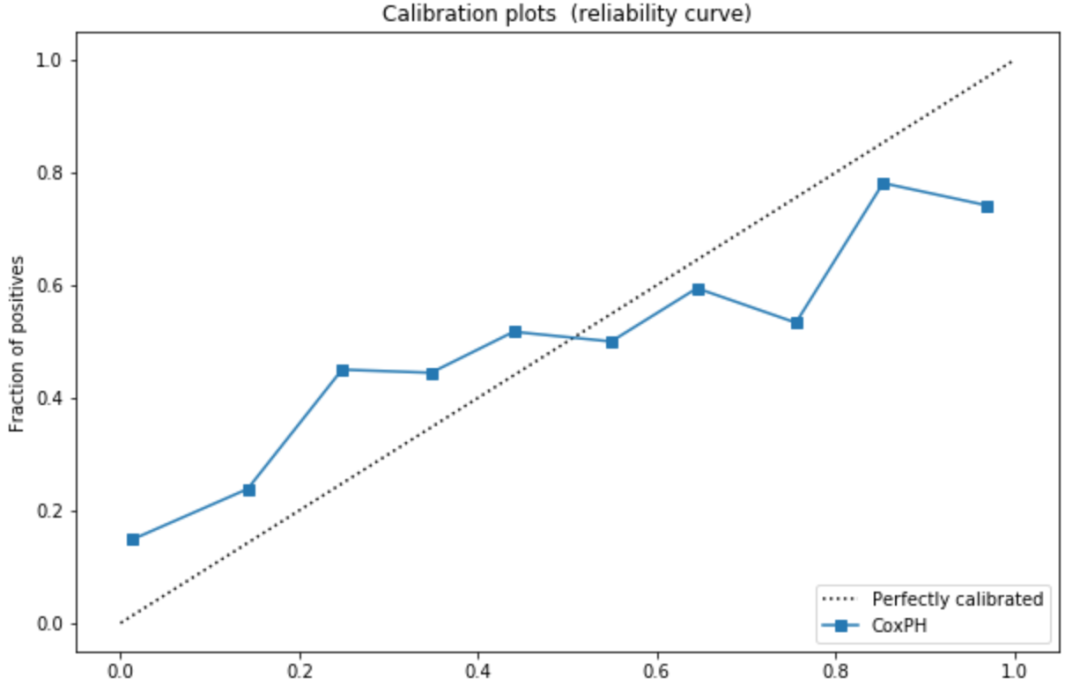brier_score_loss(
cph_test['Churn_Yes'], 1 -
np.array(cph.predict_survival_function(cph_test).loc), pos_label=1
)



loss_dict = {}
for i in range(1,73):
score = brier_score_loss(
cph_test['Churn_Yes'], 1 -
np.array(cph.predict_survival_function(cph_test).loc[i]),
pos_label=1 )
loss_dict[i] = [score]

loss_df = pd.DataFrame(loss_dict).T

fig, ax = plt.subplots()
ax.plot(loss_df.index, loss_df)
ax.set(xlabel='Prediction Time', ylabel='Calibration Loss', title='Cox PH Model Calibration Loss / Time')
ax.grid()

plt.show()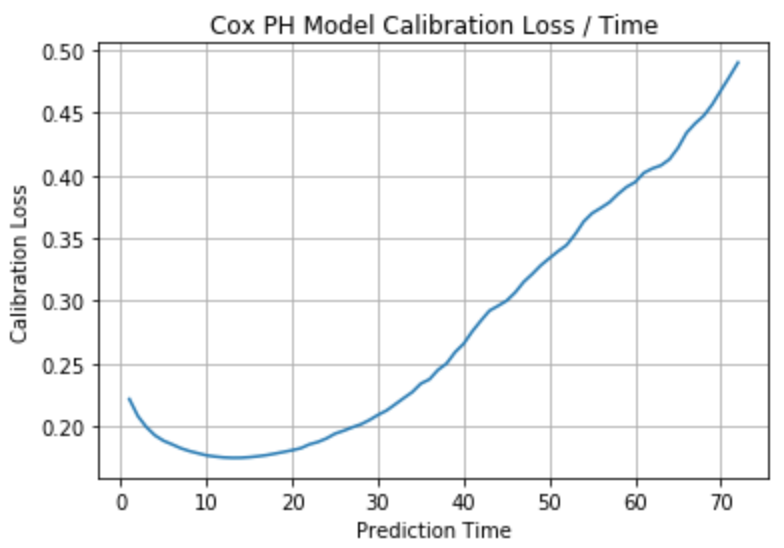loss_df.columns = ['loss']
temp_df = actions.reset_index().set_index('PaymentMethod_Credit card (automatic)').join(loss_df)
temp_df = temp_df.set_index('index')
actions['CreditCard Lower'] = temp_df['CreditCard Diff'] - (temp_df['loss'] * temp_df['CreditCard Diff'])
actions['CreditCard Upper'] = temp_df['CreditCard Diff'] + (temp_df['loss'] * temp_df['CreditCard Diff'])
temp_df = actions.reset_index().set_index('PaymentMethod_Bank transfer (automatic)').join(loss_df)
temp_df = temp_df.set_index('index')
actions['BankTransfer Lower'] = temp_df['BankTransfer Diff'] - (.5 * temp_df['loss'] * temp_df['BankTransfer Diff']) actions['BankTransfer Upper'] = temp_df['BankTransfer Diff'] + (.5 * temp_df['loss'] * temp_df['BankTransfer Diff'])

temp_df = actions.reset_index().set_index('Contract_One year').join(loss_df)
temp_df = temp_df.set_index('index')
actions['1yrContract Lower'] = temp_df['1yrContract Diff'] - (.5 * temp_df['loss'] * temp_df['1yrContract Diff']) actions['1yrContract Upper'] = temp_df['1yrContract Diff'] + (.5 * temp_df['loss'] * temp_df['1yrContract Diff'])

temp_df = actions.reset_index().set_index('Contract_Two year').join(loss_df)
temp_df = temp_df.set_index('index')
actions['2yrContract Lower'] = temp_df['2yrContract Diff'] - (.5 * temp_df['loss'] * temp_df['2yrContract Diff']) actions['2yrContract Upper'] = temp_df['2yrContract Diff'] + (.5 * temp_df['loss'] * temp_df['2yrContract Diff'])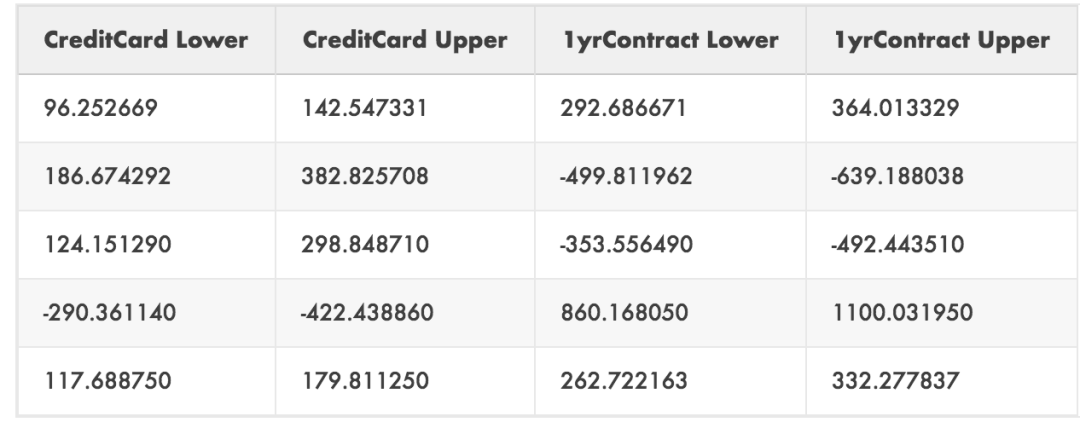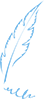END07-08

#### Python数据分析-用户流失预测实战

07-2607-253203

#### 用户流失预测——基于逻辑回归模型以及Python代码实现

03-121854

#### 项目▍用Python的Sklearn库预测电信客户流失分析

03-012028

#### 实战 | 电信客户流失分析与预测

03-228658

#### 数据分析：留存率曲线拟合

12-26348

#### 面试时，遇到决策树相关的问题怎么办？看完下面的30道题，你应该就心里有底了...

03-224523

#### 机器学习项目实战之用户流失预警

08-24834

#### 实战-----用户流失预警

05-237877

#### 机器学习实战---用户流失预测

05-233468

#### 利用python进行分类-预测顾客流失(简版)

03-141万+

#### [机器学习实战]使用 scikit-learn 预测用户流失

08-242万+

#### python数据分析：客户价值分析案例实战

05-28584

#### 利用Python进行电商网站用户行为分析

06-2688

#### 狼羽：为什么说用户留存率决定你的生死

10-104677

#### Python用户消费行为分析实例

11-191553

#### C/C++学习指南全套教程

09-11©️2020 CSDN 皮肤主题: 大白 设计师: CSDN官方博客点击重新获取扫码支付1.余额是钱包充值的虚拟货币，按照1:1的比例进行支付金额的抵扣。
2.余额无法直接购买下载，可以购买VIP、C币套餐、付费专栏及课程。余额充值